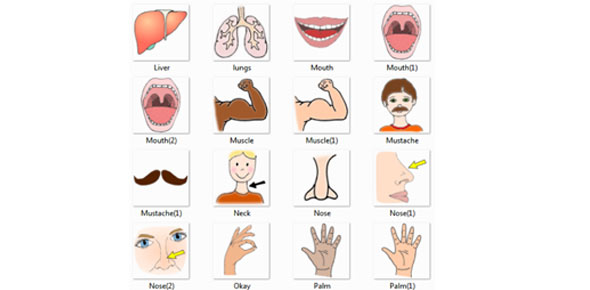# Left Handed Vs. Right Handed

Approved & Edited by ProProfs Editorial Team
At ProProfs Quizzes, our dedicated in-house team of experts takes pride in their work. With a sharp eye for detail, they meticulously review each quiz. This ensures that every quiz, taken by over 100 million users, meets our standards of accuracy, clarity, and engagement.
| Written by FaiqahFiqah
F
FaiqahFiqah
Community Contributor
Total Contribution - 1 | Total attempts - 368
Questions: 8 | Attempts: 368Settings• 1.

• A.

5000

• B.

4100

• C.

0

• D.

6000

B. 4100
Explanation
The question asks for the total after performing a series of additions. Starting with 1000, we add 40 to get 1040. Then we add another 1000 to get 2040. Next, we add 30 to get 2070. We add another 1000 to get 3070. Then we add 20 to get 3090. We add another 1000 to get 4090. Finally, we add 10 to get a total of 4100. Therefore, the correct answer is 4100.

Rate this question:

• 2.

### A farmer has 20 sheeps, and all but 8 die. How many are left?

• A.

0

• B.

12

• C.

8

• D.

20

C. 8
Explanation
The question states that all but 8 of the sheep die. This means that 12 of the sheep die, leaving only 8 sheep remaining. Therefore, the correct answer is 8.

Rate this question:

• 3.

### If 2 = 6   3 = 12   4 = 20   5 = 30   6 = 42   9 = ?

• A.

9

• B.

1

• C.

90

• D.

81

C. 90
Explanation
The pattern in the given sequence is that each number is multiplied by its position in the sequence. For example, 2 is multiplied by 2, 3 is multiplied by 3, and so on. Therefore, 9 would be multiplied by 9, resulting in 81. However, since 81 is not one of the given options, the correct answer must be the next number in the sequence, which is 90.

Rate this question:

• 4.

### Which of the following is suitable for the next pattern?

• A.

A

• B.

B

• C.

C

• D.

D

• E.

E

C. C
Explanation
The pattern in the given sequence is alphabetical order. The letters are arranged in ascending order, starting from A. Therefore, the next letter in the sequence should be the letter immediately after E, which is F. However, F is not given as an option. Among the given options, the letter that comes after E in alphabetical order is C. Therefore, C is the most suitable option for the next pattern.

Rate this question:

• 5.

### Which one does not rhyme with ballet?

• A.

Billet

• B.

Chalet

• C.

Bouquet

• D.

Wallet

• E.

A and D

E. A and D
Explanation
The words "billet" and "wallet" do not rhyme with "ballet." Rhyming words have similar sounds at the end, but "billet" and "wallet" have different sounds. On the other hand, "chalet" and "bouquet" both have similar sounds at the end, making them potential rhyming words for "ballet." Therefore, the correct answer is A and D.

Rate this question:

• 6.

### Which of the following can be rearranged to form a six letter word?

• A.

S A N I B S

• B.

B I N T S A

• C.

S R A T Z E

• D.

T A T T O S

A. S A N I B S
Explanation
The letters "S A N I B S" can be rearranged to form the word "B A S I N S".

Rate this question:

• 7.

### Which of the pattern is suitable to replace the question mark?

• A.

1

• B.

2

• C.

3

• D.

4

B. 2
Explanation
Based on the given pattern, the numbers are increasing by 1 from left to right. The first number is 1, the second number is 2, and so on. Therefore, the number that is suitable to replace the question mark is 2.

Rate this question:

• 8.

### How many faces do you see?

• A.

0

• B.

10

• C.

11

• D.

4Back to top